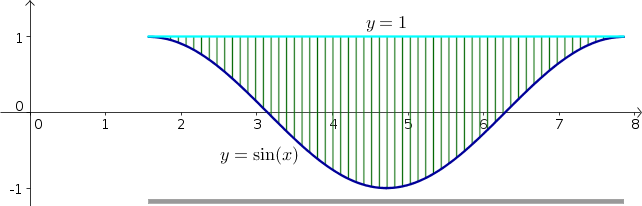# Math Insight

### Image: Change order of integration example region with sinusoid, y firstExample region illustrating process of changing the order of integration in double integrals, shown with $y$ as the inner integral. For a given value of $x$, $y$ ranges from the curve $y=\sin x$ (blue) to the line $y=1$ (cyan), as illustrated by the vertical hashing over the region. The total range of $x$ over the region is from $\pi/2$ to $5\pi/2$, as illustrated by the gray bar at the bottom.

Source image file: double_integral_change_order_example_sin_y.ggb
Source image type: Geogebra